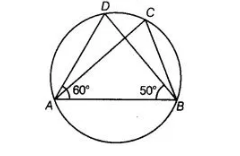# In figure, if ∠DAB = 60° ,`
Question:

In figure, if $\angle D A B=60^{\circ}, \angle A B D=50^{\circ}$, then $\angle A C B$ is equal to(a) 60°

(b) 50°

(c)70°

(d) 80°

Thinking Process

Use the theorem that angles in the same segment of a circle are equal and further simplify it.

Solution:

(c) Given, ∠DAB = 60°, ∠ABD = 50°

[angles in same segment of a circle are equal]

In ΔABD, ∠ABD + ∠ADB + ∠DAB = 180° [by angle sum property of a triangle]

50°+ ∠ADB + 60° = 180°

=> ∠ADB = 180° – 110° = 70°

$\Rightarrow \angle \mathrm{ADB}=180^{\circ}-110^{\circ}=70^{\circ}$